Consider allowing JavaScript. Otherwise, you have to be proficient in readingsince formulas will not be rendered. Furthermore, the table of contents in the left column for navigation will not be available and code-folding not supported. Sorry for the inconvenience.

Examples in this article were generated with4.2.1 by the package PowerTOST.1 See also the README on GitHub for an overview.

• The right-hand badges give the ‘level’ of the respective section.

1. Basics about sample size methodology – requiring no or only limited statistical expertise.

1. These sections are the most important ones. They are – hopefully – easily comprehensible even for novices.

1. A somewhat higher knowledge of statistics and/or R is required. May be skipped or reserved for a later reading.

1. An advanced knowledge of statistics and/or R is required. Not recommended for beginners in particular.

1. If you are not a neRd or statistics afficionado, skipping is recommended. Suggested for experts but might be confusing for others.
• Click to show / hide R code.
Abbreviation Meaning
$$\small{\alpha}$$ Nominal level of the test, probability of Type I Error (patient’s risk)
$$\small{\beta}$$ Probability of Type II Error (producer’s risk)
BE Bioequivalence
$$\small{CV_\textrm{w}}$$ Within-subject Coefficient of Variation
$$\small{\Delta}$$ Clinically relevant difference
$$\small{H_0}$$ Null hypothesis
$$\small{H_1}$$ Alternative hypothesis (also $$\small{H_\textrm{a}}$$)
$$\small{\mu_\textrm{T}/\mu_\textrm{R}}$$ True T/R-ratio
$$\small{\pi}$$ Prospective power, where $$\small{\pi=1-\beta}$$
$$\small{\theta_1,\;\theta_2}$$ Fixed lower and upper limits of the acceptance range (BE margins)

# Introduction

What is a significant treatment effect and do we have to care about one?

Sometimes regulatory assessors ask for the ‘justification’ of a significant treatment in an equivalence trial.

I will try to clarify why such a justification is futile and – a bit provocative – asking for one demonstrates a lack of understanding the underlying statistical concepts.

## Preliminaries

A basic knowledge of R is required. To run the scripts at least version 1.4.3 (2016-11-01) of PowerTOST is suggested. Any version of R would likely do, though the current release of PowerTOST was only tested with version 4.1.3 (2022-03-10) and later.
All scripts were run on a Xeon E3-1245v3 @ 3.40GHz (1/4 cores) 16GB RAM with R 4.2.1 on Win­dows 7 build 7601, Service Pack 1, Universal C Runtime 10.0.10240.16390.

The examples deal with the 2×2×2 crossover design ($$\small{\textrm{TR}|\textrm{RT}}$$)2 but the concept is applicable to any kind of cross­over (Higher-Order, replicate designs) or parallel designs assessed for equivalence.

previous section ↩︎

## Terminology

In order to get prospective power (and hence, a sample size), we need five values:

1. The level of the test $$\small{\alpha}$$ (in BE commonly 0.05),
2. the BE-margins (commonly 0.80 – 1.25),
3. the desired (or target) power $$\small{\pi}$$,
4. the variance (commonly expressed as a coefficient of variation), and
5. the deviation of the test from the reference treatment.

1 – 2 are fixed by the agency,
3 is set by the sponsor (commonly to 0.80 – 0.90), and
4 – 5 are just (uncertain!) assumptions.

In other words, obtaining a sample size is not an exact calculation but always just an estimation.

Realization: Observations (in a sample) of a random variable (of the population).

It is extremely unlikely that all assumptions will be exactly realized in a particular study. If the realized values differ from the assumptions (i.e., T less deviating from R, and/or lower CV, and/or fewer dropouts than anticipated), power and hence, the chance to observe a statistically significant treatment effect increases.

previous section ↩︎

# Hypotheses

Let us first recap the statistical hypotheses in bioequivalence.

• The confidence interval inclusion approach:

$H_0:\frac{\mu_\textrm{T}}{\mu_\textrm{R}}\ni\left\{\theta_1,\theta_2\right\}\:vs\:H_1:\theta_1<\frac{\mu_\textrm{T}}{\mu_\textrm{R}}<\theta_2\tag{1}$

• The ‘Two One-Sided Tests’ (TOST) Procedure:3

$\begin{matrix}\tag{2} H_\textrm{0L}:\frac{\mu_\textrm{T}}{\mu_\textrm{R}}\leq\theta_1\:vs\:H_\textrm{1L}:\frac{\mu_\textrm{T}}{\mu_\textrm{R}}>\theta_1\\ H_\textrm{0U}:\frac{\mu_\textrm{T}}{\mu_\textrm{R}}\geq\theta_2\:vs\:H_\textrm{1U}:\frac{\mu_\textrm{T}}{\mu_\textrm{R}}<\theta_2 \end{matrix}$

The lower and upper limits of the bioequivalence range $$\small{\{\theta_1,\theta_2\}}$$ are calculated based on the ‘clinically not relevant difference’ $$\small{\Delta}$$ $\left\{\theta_1=100\,(1-\Delta),\,\theta_2=100\,(1-\Delta)^{-1}\right\}\tag{3}$ Commonly $$\small{\Delta}$$ is set to 0.20. For narrow therapeutic index drugs (the EMA and other jurisdictions) $$\small{\Delta}$$ is set to 0.10, and for $$\small{C_\textrm{max}}$$ (Russian Federation, the EEU, South Africa) $$\small{\Delta}$$ is set to 0.25. We obtain: $\begin{matrix}\tag{4} \left\{\theta_1=80.00\%,\,\theta_2=125.00\%\right\}\\ \left\{\theta_1=90.00\%,\,\theta_2=111.1\dot{1}\%\right\}\\ \left\{\theta_1=75.00\%,\,\theta_2=133.3\dot{3}\%\right\} \end{matrix}$

$$\small{(2)}$$ is operationally identical to $$\small{(1)}$$. From the TOST procedure we obtain two $$\small{p}$$-values (where $$\small{H_0}$$ is not rejected if $$\small{\max}\{p_\textrm{L},p_\textrm{U}\}>\alpha$$). It is of historical interest because only $$\small{(1)}$$ is mentioned in regulatory guidelines.

As long as the $$\small{100\,(1-2\,\alpha)}$$ confidence interval lies entirely within pre-specified limits $$\small{\{\theta_1,\theta_2\}}$$, the null hypothesis of inequivalence in $$\small{(1)}$$ is rejected and the alternative hypothesis of equivalence in $$\small{(1)}$$ is accepted.
Neither the point estimate $$\small{\theta_0}$$ nor the width of the CI, as well as post hoc power play any role in this decision.

Since $$\small{(1)}$$ and $$\small{(2)}$$ are operationally equivalent, it follows that if one of the $$\small{p}$$ values of $$\small{(2)}$$ < $$\small{\alpha}$$, the $$\small{100\,(1-2\,\alpha)}$$ CI does not include 100%.

In such a case treatments differ statistically significant, although this difference is not clinically relevant.

Asking for the ‘justification’ of a statistical significant treatment difference contradicts the accepted standard by $$\small{(1)}$$ – as laid down in guidances since the early 1990s.4 5 6 As an aside, this standard has not changed ever since.

With a sufficiently large sample size any treatment with a $$\small{\theta_0\neq100\%}$$ will show a statistically significant difference.7

# Models

## Fixed effects

Most agencies (like the EMA) require an ANOVA of $$\small{\log_{e}}$$ transformed responses, i.e., a linear model where all effects are fixed.

In R

model <- lm(logPK ~ sequence + subject %in% sequence +
period + treatment, data = data)
coef(model)[["treatmentT"]]
confint(model, "treatmentT", level = 0.9)
In SAS
PROC GLM
data = data;
class subject period sequence treatment;
model logPK = sequence subject(sequence)
period treatment;
estimate 'T-R' treatment -1 1 / CL alpha = 0.1;
run;


In Phoenix WinNonlin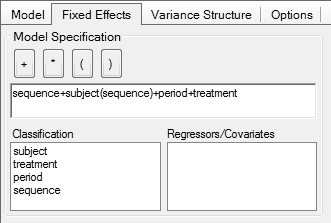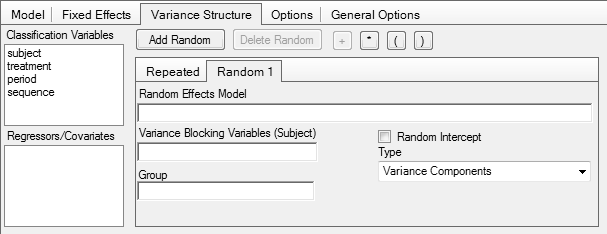## Mixed effects

The FDA and Health Canada require a mixed-effects model where sequence, period, and treatment are fixed effects and subject(sequence) is a random effect.

In R

library(nlme)
model <- lme(logPK ~ sequence + period + treatment,
random = ~ 1 | subject, data = data)
intervals(model, which = "fixed",
level= 1 - 2 * 0.05)[]["treatmentT", c(1, 3)]
In SAS
PROC MIXED
data = data;
class subject period sequence treatment;
model logPK = sequence subject(sequence)
period treatment;
estimate 'T-R' treatment -1 1 / CL alpha = 0.1;
run;


In Phoenix WinNonlin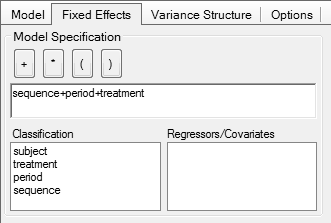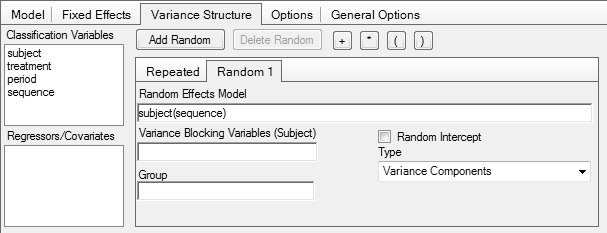# Examples

Throughout the examples I’m dealing with studies in a 2×2×2 Crossover Design. Of course, the same logic is applicable for any other as well.

library(PowerTOST) # attach it to run the examples
balance <- function(n, seq = 2) {
return(as.integer(seq * (n %/% seq + as.logical(n %% seq))))
}
nadj <- function(n, do, seq) {
return(as.integer(balance(n / (1 - do), seq)))
}

## Single endpoint

### Example 1

Say, the assumed CV was 15%, the T/R-ratio 0.95, and we planned the study for power ≥ 90% and an anticipated a dropout-rate of 15%. More subjects than estimated were dosed (quite often the management decides). In a small survey a whopping 37% of respondents reported that.8

CV     <- 0.15
target <- 0.90
do     <- 0.15
theta0 <- pe <- TR <- 0.95
n      <- sampleN.TOST(CV = CV, targetpower = target,
theta0 = theta0,
print = FALSE)[["Sample size"]]
ns     <- n:(n*2.5)
res1   <- data.frame(n = ns, CL.lo = NA, CL.hi = NA,
p.lo = NA, p.hi = NA,
post.hoc = NA)
# as planned
for (i in seq_along(ns)) {
res1[i, 2:3] <- 100*CI.BE(CV = CV, pe = pe,
n = ns[i])
res1[i, 4:5] <- suppressMessages(
pvalues.TOST(CV = CV, pe = pe,
n = ns[i]))
res1[i, 6]   <- suppressMessages(
power.TOST(CV = CV, n = ns[i],
theta0 = theta0))
}
windows(width = 4.5, height = 4.5)
par(mar = c(4.1, 4, 0, 0), cex.axis = 0.9)
plot(ns, rep(100, length(ns)), ylim = c(80, 125),
type = "n", axes = FALSE, log = "y",
xlab = "sample size",
ylab = "T/R-ratio  (90% CI)")
axis(1, at = seq(n, tail(ns, 1), 6))
axis(2, at = c(80, 90, 100, 110, 120, 125), las = 1)
grid(nx = NA, ny = NULL)
abline(v = seq(n, tail(ns, 1), 6), col = "lightgray", lty = 3)
abline(h = c(80, 100, 125), lty = 2,
col = c("red", "black", "red"))
abline(v = head(res1$n[res1$CL.hi < 100], 1), lty = 2)
segments(x0 = ns, x1 = tail(ns, 1),
y0 = 100*TR, col = "blue")
lines(res1$n, res1$CL.lo, type = "s", col = "blue", lwd = 2)
lines(res1$n, res1$CL.hi, type = "s", col = "blue", lwd = 2)
box()
par(op)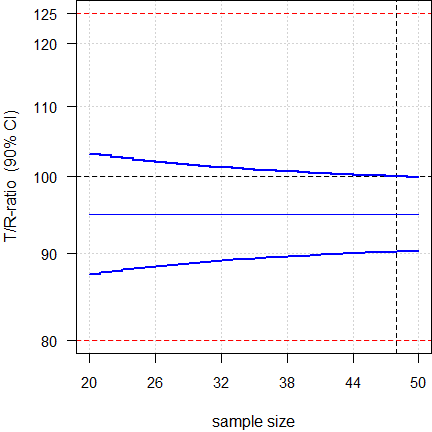Fig. 1 Realized values = assumptions.

We face a significant treatment effect with ≥ 48 subjects (upper confidence limit ≤ 99.98%).

### Example 2

Similar to the previous example but the management was more relaxed (24 subjects dosed).
This time the T/R-ratio turned out to be worse (0.92 instead of the assumed 0.95).

theta0 <- 0.95
target <- 0.90
do     <- 0.15
TR     <- 0.92
n      <- sampleN.TOST(CV = CV, targetpower = target,
theta0 = theta0,
print = FALSE)[["Sample size"]]
n.hi   <- balance(n.adj * 1.2, 2)
ns     <- n:n.hi
res2   <- data.frame(n = ns, CL.lo = NA, CL.hi = NA,
p.lo = NA, p.hi = NA,
post.hoc = NA)
for (i in seq_along(ns)) {
res2[i, 2:3] <- 100*CI.BE(CV = CV, pe = TR,
n = ns[i])
res1[i, 4:5] <- suppressMessages(
pvalues.TOST(CV = CV, pe = TR,
n = ns[i]))
res1[i, 6]   <- suppressMessages(
power.TOST(CV = CV, n = ns[i],
theta0 = TR))
}
windows(width = 4.5, height = 4.5)
par(mar = c(4.1, 4, 0, 0), cex.axis = 0.9)
plot(ns, rep(100, length(ns)), ylim = c(80, 125),
type = "n", axes = FALSE, log = "y",
xlab = "sample size",
ylab = "T/R-ratio  (90% CI)")
axis(1, at = seq(n, tail(ns, 1), 2))
axis(2, at = c(80, 90, 100, 110, 120, 125), las = 1)
grid(nx = NA, ny = NULL)
abline(v = seq(n, tail(ns, 1), 2), col = "lightgray", lty = 3)
abline(h = c(80, 100, 125), lty = 2,
col = c("red", "black", "red"))
abline(v = head(res2$n[res2$CL.hi < 100], 1), lty = 2)
segments(x0 = ns, x1 = tail(ns, 1),
y0 = 100*TR, col = "blue")
lines(res2$n, res2$CL.lo, type = "s", col = "blue", lwd = 2)
lines(res2$n, res2$CL.hi, type = "s", col = "blue", lwd = 2)
box()
par(op)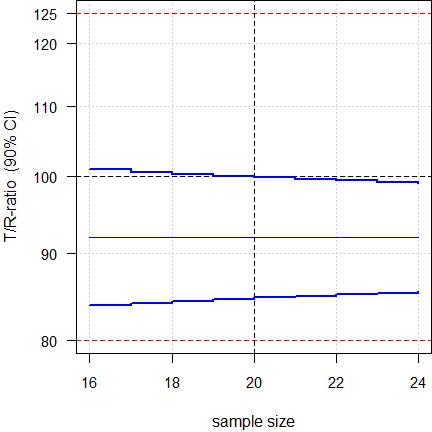Fig. 2 Worse T/R-ratio.

We face a significant treatment effect with ≥ 20 subjects (upper confidence limit ≤ 99.84%).

### Example 3

We had to deal with a drug with low variability. The assumed CV was 10%, the T/R-ratio 0.95, and we planned the study for power ≥ 80%. Theoretically we would need only eight [sic] subjects but the minimum sample size according to the guidelines is twelve. We adjusted the sample size for an anticipated a dropout-rate of 15%.
The T/R-ratio turned out to be slightly worse (0.935 instead of the assumed 0.95).

CV     <- 0.10
target <- 0.80
theta0 <- 0.95
TR     <- 0.935
do     <- 0.15
n      <- sampleN.TOST(CV = CV, targetpower = target,
theta0 = theta0,
print = FALSE)[["Sample size"]]
if (n < 12) n <- 12      # acc. to GL
res3   <- data.frame(n = ns, CL.lo = NA, CL.hi = NA,
p.lo = NA, p.hi = NA,
post.hoc = NA)
for (i in seq_along(ns)) {
res3[i, 2:3] <- 100*CI.BE(CV = CV, pe = TR,
n = ns[i])
res1[i, 4:5] <- suppressMessages(
pvalues.TOST(CV = CV, pe = TR,
n = ns[i]))
res3[i, 6]   <- suppressMessages(
power.TOST(CV = CV, n = ns[i],
theta0 = TR))
}
windows(width = 4.5, height = 4.5)
par(mar = c(4.1, 4, 0, 0), cex.axis = 0.9)
plot(ns, rep(100, length(ns)), ylim = c(80, 125),
type = "n", axes = FALSE, log = "y",
xlab = "sample size",
ylab = "T/R-ratio  (90% CI)")
axis(1, at = ns)
axis(2, at = c(80, 90, 100, 110, 120, 125), las = 1)
grid(nx = NA, ny = NULL)
abline(v = ns, col = "lightgray", lty = 3)
abline(h = c(80, 100, 125), lty = 2,
col = c("red", "black", "red"))
abline(v = head(res3$n[res3$CL.hi < 100], 1), lty = 2)
segments(x0 = ns, x1 = tail(ns, 1),
y0 = 100*TR, col = "blue")
lines(res3$n, res3$CL.lo, type = "s", col = "blue", lwd = 2)
lines(res3$n, res3$CL.hi, type = "s", col = "blue", lwd = 2)
box()
par(op)Fig. 3 Low CV, worse T/R-ratio.

Already with 14 subjects we face a significant treatment effect (upper confidence limit 99.998%). This ‘nasty’ value will disappear due to rounding but will remain in the output of the ANOVA.

Drugs with a low CV regularly show a significant treatment effect, since following the guidelines leads to ‘overpowered’ studies. With the minimum twelve subjects according to the guidelines we will have a post hoc power of 97.2% (though we planned only for 80%).

## Multiple endpoints

Commonly equivalence of more than one endpoint has to be demonstrated.
In bioequivalence the pharmacokinetic metrics $$\small{C_\textrm{max}}$$ and $$\small{AUC_{0-\textrm{t}}}$$ are mandatory (in some jurisdictions like the FDA additionally $$\small{AUC_{0-\infty}}$$).

We don’t have to worry about multiplicity issues (inflated Type I Error) since if all tests must pass at level $$\alpha$$, we are protected by the intersection-union principle.9 10

We design the study always for the worst case combination, i.e., based on the PK metric requiring the largest sample size.

### Example 1

The assumed CV of $$\small{C_\textrm{max}}$$ is 25% and the one of $$\small{AUC}$$ is lower (say the variance ratio is 0.70). We assume a T/R-ratio of 0.95 for both, aiming at power ≥ 80%. The anticipated dropout-rate is 10%.

The supportive function opt() provides extreme point estimates of $$\small{AUC}$$ which will still give our target power (only the lower one is given in the 4th column). If this value is realized in the study, its upper confidence limit will not include 100% if we have not at least two droputs.

opt <- function(x) {
suppressMessages(
power.TOST(theta0 = x, CV = res4$CV, n = n.est)) - target } metrics <- c("Cmax", "AUC") ratio <- 0.70 # variance ratio (AUC / Cmax) CV.Cmax <- 0.25 # CV of Cmax CV.AUC <- mse2CV(CV2mse(CV.Cmax)*ratio) CV <- signif(c(CV.Cmax, CV.AUC)) theta0 <- 0.95 # both metrics target <- 0.80 # target (desired) power do.rate <- 0.10 # anticipated dropout-rate 10% res4 <- data.frame(metric = metrics, theta0 = theta0, CV = CV, n = NA, power1 = NA, nadj = NA, power2 = NA) # sample sizes for both metrics # study sample size based one the one with # higher CV and adjusted for the dropout-rate for (i in 1:nrow(res4)) { res4[i, 4:5] <- sampleN.TOST(CV = CV[i], theta0 = theta0, targetpower = target, print = FALSE)[7:8] } res4$nadj   <- nadj(max(res4$n), do.rate, 2) res4$power1 <- res4$power1 for (i in 1:nrow(res4)) { res4[i, 7] <- power.TOST(CV = CV[i], theta0 = theta0, n = res4$nadj[i])
}
res4[, c(5, 7)] <- signif(res4[, c(5, 7)], 4)
names(res4)[c(5, 7)] <- c("pwr (n)", "pwr (nadj)")
res5   <- data.frame(n = max(res4$nadj):max(res4$n),
PE = theta0, CL.hi1 = NA,
PE.lo = NA, CL.hi2 = NA, power = NA)
for (i in 1:nrow(res5)) {
n.est <- res5$n[i] if (theta0 < 1) { res5$PE.lo[i] <- uniroot(opt, tol = 1e-8,
interval =
c(0.80 + 1e-4,
theta0))$root }else { res5$PE.lo[i] <- uniroot(opt, tol = 1e-8,
interval =
c(theta0,
1.25 - 1e-4))$root } res5[i, 3] <- CI.BE(CV = CV, pe = theta0, n = res5$n[i])[["upper"]]
res5[i, 5] <- CI.BE(CV = CV,
pe = res5$PE.lo[i], n = res5$n[i])[["upper"]]
res5[i, 6] <- round(suppressMessages(
power.TOST(CV = CV, n = n.est)), 4)
}
res5[, 2:5] <- round(100*res5[, 2:5], 2)
names(res5)[c(3, 5)] <- rep("upper CL", 2)
print(res4, row.names = FALSE)
cat("AUC:\n");
print(res5, row.names = FALSE)
#  metric theta0       CV  n pwr (n) nadj pwr (nadj)
#    Cmax   0.95 0.250000 28  0.8074   32     0.8573
#     AUC   0.95 0.208208 20  0.8057   32     0.9467
# AUC:
#   n PE upper CL PE.lo upper CL  power
#  32 95   103.68 91.20    99.53 0.9467
#  31 95   103.83 91.41    99.91 0.9403
#  30 95   104.00 91.62   100.29 0.9337
#  29 95   104.17 91.85   100.71 0.9258
#  28 95   104.35 92.08   101.14 0.9176

Due to its lower CV we would need only 20 subjects for $$\small{AUC}$$. However, for $$\small{C_\textrm{max}}$$ we need 28. We perform the study in 32 subjects (adjusted for the dropout-rate). Consequently, the study is ‘overpowered’ for $$\small{AUC}$$ (~95% instead of ~81% with 20 subjects).

Such a situation is quite common and the further the CVs of pharmacokinetic metrics are apart, the more often we will face it.

In a particular study the point estimate may be even lower and/or the CV whilst the study still passes. Then we will get a significant treatment effect with more dropouts.

### Example 2

Although that is straightforward and based on elementary statistics, below an R-script to perform simulations.

Say we assume a CV of $$\small{C_\textrm{max}}$$ (25%), base the sample size on it (taking the dropout-rate into account) and the CV of $$\small{AUC}$$ is lower but unknown. How often can we expect a significant treatment effect for a range of CVs (here 25% down to 15%)?

# Cave: Long runtime!
set.seed(123456)
nsims   <- 1e4L # number of simulations
target  <- 0.80 # target power
PE      <- 0.95 # assumed PE (both metrics)
CV.Cmax <- 0.25 # assumed CV of Cmax
CV.AUC  <- c(0.25, 0.20, 0.15)
do.rate <- 0.1  # anticipated dropout-rate (10%)
CV.do   <- 0.15 # assumed CV of the dropout-rate (15%)
tmp     <- sampleN.TOST(CV = CV.Cmax, theta0 = PE,
targetpower = target,
details = FALSE, print = FALSE)
n.des   <- tmp[["Sample size"]]
if (n.des >= 12) {
power.Cmax <- tmp[["Achieved power"]]
} else {# GL!
n.des <- 12
power.Cmax <- power.TOST(CV = CV.Cmax, theta0 = PE, n = n.des)
}
power.AUC <- numeric()
for (j in seq_along(CV.AUC)) {
power.AUC[j] <- power.TOST(CV = CV.AUC[j], theta0 = PE, n = n.des)
}
res.Cmax  <- data.frame(CV = rep(NA, nsims), n = NA, PE = NA,
lower = NA, upper = NA, BE = FALSE,
signif = FALSE)
post.Cmax <- data.frame(sim = 1:nsims)
res.AUC   <- data.frame(CV.ass = rep(CV.AUC, each = nsims),
CV = rep(NA, nsims), n = NA, PE = NA,
lower = NA, upper = NA, BE = FALSE,
signif = FALSE)
post.AUC  <- data.frame(CV.ass = rep(CV.AUC, each = nsims),
sim =1:nsims*length(CV.AUC))
for (j in 1:nsims) {
do                <- rlnorm(1, meanlog = log(do.rate) - 0.5*CV2mse(CV.do),
sdlog = sqrt(CV2mse(CV.do)))
res.Cmax$n[j] <- as.integer(round(n.des * (1 - do))) res.Cmax$CV[j]    <- mse2CV(CV2mse(CV.Cmax) *
rchisq(1, df = res.Cmax$n[j] - 2)/(res.Cmax$n[j] - 2))
res.Cmax$PE[j] <- exp(rnorm(1, mean = log(PE), sd = sqrt(0.5 / res.Cmax$n[j]) *
sqrt(CV2mse(CV.Cmax))))
res.Cmax[j, 4:5]  <- round(CI.BE(CV = res.Cmax$CV[j], pe = res.Cmax$PE[j],
n = res.Cmax$n[j]), 4) if (res.Cmax$lower[j] >= 0.80 & res.Cmax$upper[j] <= 1.25) { res.Cmax$BE[j] <- TRUE
if (res.Cmax$lower[j] > 1 | res.Cmax$upper[j] < 1)
res.Cmax$signif[j] <- TRUE } } i <- 0 for (k in seq_along(CV.AUC)) { for (j in 1:nsims) { i <- i + 1 res.AUC$n[i]     <- res.Cmax$n[j] res.AUC$CV[i]    <- mse2CV(CV2mse(CV.AUC[k]) *
rchisq(1, df = res.AUC$n[i] - 2)/(res.AUC$n[i] - 2))
res.AUC$PE[i] <- exp(rnorm(1, mean = log(PE), sd = sqrt(0.5 / res.AUC$n[i]) *
sqrt(CV2mse(CV.AUC[k]))))
res.AUC[i, 5:6]  <- round(CI.BE(CV = res.AUC$CV[i], pe = res.AUC$PE[i],
n = res.AUC$n[i]), 4) if (res.AUC$lower[i] >= 0.80 & res.AUC$upper[i] <= 1.25) { res.AUC$BE[i] <- TRUE
if (res.AUC$lower[i] > 1 | res.AUC$upper[i] < 1)
res.AUC$signif[i] <- TRUE } } } passed.Cmax <- sum(res.Cmax$BE)
passed.AUC  <- numeric(length(CV.AUC))
for (j in seq_along(CV.AUC)) {
passed.AUC[j] <- sum(res.AUC$BE[res.AUC$CV.ass == CV.AUC[j]])
}
txt <- paste("Assumed CV (Cmax)    :", sprintf("%.4f", CV.Cmax),
"\nAssumed CVs (AUC)    :", paste(sprintf("%.4f", CV.AUC),
collapse = ", "),
"\nAssumed PE           :", sprintf("%.4f", PE),
"\nTarget power         :", sprintf("%.4f", target),
"\nSample size          :", n.des, "(based on Cmax)",
"\nAchieved power (Cmax):", sprintf("%.4f", power.Cmax),
"\nAchieved powers (AUC):", paste(sprintf("%.4f", power.AUC),
collapse = ", "),
sprintf("(anticip. dropout-rate %g)", do.rate),
"\n ", formatC(nsims, format = "d", big.mark = ","),
"simulated 2\u00D72\u00D72 studies",
"\n  n:", min(res.Cmax$n), "\u2013", max(res.Cmax$n),
sprintf("(median %g)", median(res.Cmax$n)), "\n Cmax", sprintf("(%.4f)", CV.Cmax), "\n CV :", sprintf("%6.4f \u2013 %6.4f", min(res.Cmax$CV), max(res.Cmax$CV)), sprintf("(median %7.4f)", exp(median(log(res.Cmax$CV)))),
"\n    PE       :",
sprintf("%6.4f \u2013 %6.4f", min(res.Cmax$PE), max(res.Cmax$PE)),
sprintf("(g. mean%7.4f)", exp(mean(log(res.Cmax$PE)))), "\n 100% not within CI (stat. significant):", sprintf("%5.2f%%", 100*sum(res.Cmax$signif)/passed.Cmax), "\n")
for (j in seq_along(CV.AUC)) {
txt <- paste(txt, "  AUC", sprintf("(%.4f)", CV.AUC[j]),
"\n    CV       :",
sprintf("%6.4f \u2013 %6.4f",
min(res.AUC$CV[res.AUC$CV.ass == CV.AUC[j]]),
max(res.AUC$CV[res.AUC$CV.ass == CV.AUC[j]])),
sprintf("(median %7.4f)",
exp(median(log(res.AUC$CV[res.AUC$CV.ass == CV.AUC[j]])))),
"\n    PE       :",
sprintf("%6.4f \u2013 %6.4f",
min(res.AUC$PE[res.AUC$CV.ass == CV.AUC[j]]),
max(res.AUC$PE[res.AUC$CV.ass == CV.AUC[j]])),
sprintf("(g. mean%7.4f)",
exp(mean(log(res.AUC$PE[res.AUC$CV.ass == CV.AUC[j]])))),
"\n    100% not within CI (stat. significant):",
sprintf("%5.2f%%",
100*sum(res.AUC$signif[res.AUC$CV.ass == CV.AUC[j]])/passed.AUC[j]),
"\n")
}
cat(txt)
# Assumed CV (Cmax)    : 0.2500
# Assumed CVs (AUC)    : 0.2500, 0.2000, 0.1500
# Assumed PE           : 0.9500
# Target power         : 0.8000
# Sample size          : 28 (based on Cmax)
# Achieved power (Cmax): 0.8074
# Achieved powers (AUC): 0.8074, 0.9349, 0.9946
# Dosed                : 32 (anticip. dropout-rate 0.1)
#   10,000 simulated 2×2×2 studies
#   n: 23 – 26 (median 25)
#   Cmax (0.2500)
#     CV       : 0.1199 – 0.4127 (median  0.2468)
#     PE       : 0.8314 – 1.0736 (g. mean 0.9502)
#     100% not within CI (stat. significant):  2.30%
#    AUC (0.2500)
#     CV       : 0.1248 – 0.4099 (median  0.2462)
#     PE       : 0.8373 – 1.0814 (g. mean 0.9491)
#     100% not within CI (stat. significant):  2.70%
#    AUC (0.2000)
#     CV       : 0.1008 – 0.3302 (median  0.1969)
#     PE       : 0.8506 – 1.0458 (g. mean 0.9501)
#     100% not within CI (stat. significant):  7.96%
#    AUC (0.1500)
#     CV       : 0.0831 – 0.2663 (median  0.1481)
#     PE       : 0.8722 – 1.0216 (g. mean 0.9497)
#     100% not within CI (stat. significant): 19.96%

What does that mean? You name it.

### Example 3

In most jurisdictions (e.g. the EMA) reference-scaling is acceptable for $$\small{C_\textrm{max}}$$, whereas for $$\small{AUC}$$ conventional ABE has to be assessed. If $$\small{AUC}$$ is highly variable as well, the study will be ‘overpowered’ for $$\small{C_\textrm{max}}$$ and a significant treatment effect possible.

metrics <- c("Cmax", "AUC")
CV      <- c(0.45, 0.35)
theta0  <- rep(0.90, 2)
theta1  <- 0.80
theta2  <- 1 / theta1
target  <- 0.80
design  <- "2x2x4"
plan    <- data.frame(metric = metrics,
method = c("ABEL", "ABE"),
CV = CV, theta0 = theta0,
L = 100 * theta1, U = 100 * theta2,
n = NA_integer_, power = NA_real_)
for (i in 1:nrow(plan)) {
if (plan$method[i] == "ABEL") { # L and U are the expanded limits plan[i, 5:6] <- round(100*scABEL(CV = CV[i]), 2) plan[i, 7:8] <- signif( sampleN.scABEL(CV = CV[i], theta0 = theta0[i], theta1 = theta1, theta2 = theta2, targetpower = target, design = design, details = FALSE, print = FALSE)[8:9], 4) }else { plan[i, 7:8] <- signif( sampleN.TOST(CV = CV[i], theta0 = theta0[i], theta1 = theta1, theta2 = theta2, targetpower = target, design = design, print = FALSE)[7:8], 4) } } txt <- paste0("L and U are the – if applicable, expanded – BE limits.", "\nSample size based on ", plan$metric[plan$n == max(plan$n)], ".")
pwr <- power.scABEL(CV = plan$CV[plan$metric ==
plan$metric[plan$n == min(plan$n)]], theta0 = plan$theta0[plan$metric == plan$metric[plan$n == min(plan$n)]],
n = plan$n[plan$n == max(plan$n)], design = design) CI <- CI.BE(CV = plan$CV[plan$metric == plan$metric[plan$n == min(plan$n)]],
pe = plan$theta0[plan$metric ==
plan$metric[plan$n == min(plan$n)]], n = max(plan$n), design = design)
txt <- paste0(txt, "\nPower for ", plan$metric[plan$n == min(plan$n)], " with ", max(plan$n), " subjects: ",
sprintf("%.4g", pwr), ".")

if (CI[["lower"]] > 1 | CI[["upper"]] < 1) {
txt <- paste0(txt, "\nSignificant treatment effect for ",
plan$metric[plan$n == min(plan$n)], " expected.\n") } else { txt <- paste0(txt, "\nNo significant treatment effect for ", plan$metric[plan$n == min(plan$n)], " expected.\n")
}
plan[, 5]   <- sprintf("%.2f%%", plan[, 5])
plan[, 6]   <- sprintf("%.2f%%", plan[, 6])
print(plan, row.names = FALSE)
cat(txt)
#  metric method   CV theta0      L       U  n  power
#    Cmax   ABEL 0.45    0.9 72.15% 138.59% 28 0.8112
#     AUC    ABE 0.35    0.9 80.00% 125.00% 52 0.8003
# L and U are the – if applicable, expanded – BE limits.
# Sample size based on AUC.
# Power for Cmax with 52 subjects: 0.9579.
# Significant treatment effect for Cmax expected.

Let’s explore what might happen if all assumed values will be realized in the study.

res         <- data.frame(metric = metrics,
method = c("ABEL", "ABE"),
CV = CV, theta0 = theta0,
lower.CL = NA_real_,
upper.CL =  NA_real_,
n = max(plan$n), power = NA_real_) res[1, 5:6] <- CI.BE(CV = CV, pe = theta0, n = max(plan$n),
design = design)
res[1, 8]   <- power.scABEL(CV = CV, theta0 = theta0,
n = max(plan$n), design = design) res[2, 5:6] <- CI.BE(CV = CV, pe = theta0, n = max(plan$n),
design = design)
res[2, 8]   <- power.TOST(CV = CV, theta0 = theta0,
n = max(plan$n), design = design) res[, 5] <- sprintf("%.2f%%", 100 * res[, 5]) res[, 6] <- sprintf("%.2f%%", 100 * res[, 6]) res[, 8] <- sprintf("%.4g", res[, 8]) print(res, row.names = FALSE) # metric method CV theta0 lower.CL upper.CL n power # Cmax ABEL 0.45 0.9 81.55% 99.32% 52 0.9579 # AUC ABE 0.35 0.9 83.25% 97.30% 52 0.8003 Although we expected a significant treatment effect only for $$\small{C_\textrm{max}}$$, for both metrics the upper confidence limits are with 99.32% and 97.30% below 100%. Like in the other examples, the study is ‘overpowered” for $$\small{C_\textrm{max}}$$. ### Example 4 Sometimes drugs are classified as an NTID but narrowing the acceptance range is only required for one of the PK metrics. An example is tacrolimus, where the EMA recommends 90.00 – 111.11% for $$\small{AUC_{0-72}}$$ and 80.00 – 125.00% for $$\small{C_\textrm{max}}$$.11 Let’s explore a study from the literature.12 Assuming a CV of ≈11% and a T/R-ratio of 95% the authors claimed that the study was powered to 80% for the narrow limits of $$\small{AUC_{0-72}}$$ with 52 subjects.13 56 subjects were dosed and 52 completed both periods of the study. design <- "2x2x2" n <- 52 AUC <- setNames(c(1.037831, 0.974019, 1.105824, 0.1949), c("PE", "lower", "upper", "CV")) Cmax <- setNames(c(1.150745, 1.098079, 1.205938, 0.1433), c("PE", "lower", "upper", "CV")) res <- data.frame(metric = c("AUC", "Cmax"), CV = c(AUC[["CV"]], Cmax[["CV"]]), PE = c(AUC[["PE"]], Cmax[["PE"]]), lower.CL = c(AUC[["lower"]], Cmax[["lower"]]), upper.CL = c(AUC[["upper"]], Cmax[["upper"]]), theta1 = c(0.90, 0.80), theta2 = 1 / c(0.90, 0.80), p = NA, power = NA) for (i in 1:nrow(res)) { res$p[i]     <- pvalue.TOST(pe = res$PE[i], CV = res$CV[i], n = n,
theta1 = res$theta1[i]) res$power[i] <- power.TOST(CV = res$CV[i], n = n, theta1 = res$theta1[i],
theta0 = res\$PE[i], design = design)
}
res        <- res[, -c(6:7)] # remove thetas
res[, 6:7] <- round(res[, 6:7], 4)
res[, 3]   <- sprintf("%.2f%%", 100 * res[, 3])
res[, 4]   <- sprintf("%.2f%%", 100 * res[, 4])
res[, 5]   <- sprintf("%.2f%%", 100 * res[, 5])
print(res, row.names = FALSE)
#  metric     CV      PE lower.CL upper.CL      p  power
#     AUC 0.1949 103.78%   97.40%  110.58% 0.0388 0.5333
#    Cmax 0.1433 115.07%  109.81%  120.59% 0.0024 0.8986

The study passed BE for both metrics (90% CI of $$\small{AUC_{0-72}}$$ within 90.00 – 111.11% and of $$\small{C_\textrm{max}}$$ within 80.00 – 125.00%). We see a significant treatment effect for $$\small{C_\textrm{max}}$$ because its confidence interval of 109.81 – 120.59% does not include 100%.

The CVs were substantially higher than the assumed 11%. The T/R-ratio of $$\small{C_\textrm{max}}$$ was much worse than assumed and only the large sample size required for $$\small{AUC_{0-72}}$$ ‘saved’ the study from failing. Since with 110.58% the upper confidence limit of $$\small{AUC_{0-7 2}}$$ was close to the boundary of 111.11%, post hoc power was only 53.33%. Whilst not clinically relevant (see another article), IMHO, it was curageous to assume such a low variability taking results of other studies into account.

# Summary

Coming back to the questions asked in the Introduction. To repeat:

What is a significant treatment effect … ?

• It is a natural property of any test at level α powered with π.
If a study passes, it does not refute the conclusion (statistical significance ≠ clinical relevance).

… and do we have to care about one?

• Not at all.
However, if you are asked by a regulator for a ‘justification’, answer in a diplomatic way. Keep calm and stay polite.

previous section ↩︎Helmut Schütz 2022
R and PowerTOST GPL 3.0, pandoc GPL 2.0.
1st version March 18, 2021. Rendered June 27, 2022 13:53 CEST by rmarkdown via pandoc in 0.70 seconds.

Footnotes and References

1. Labes D, Schütz H, Lang B. PowerTOST: Power and Sample Size for (Bio)Equivalence Studies. Package version 1.5.4. 2022-02-21. CRAN.↩︎

2. Statisticians may be more familiar with the terminology $$\small{\textrm{AB}|\textrm{BA}}$$.
Forgive me – in bioequivalence $$\small{\textrm{TR}|\textrm{RT}}$$ is commonly used, where $$\small{\textrm{T}}$$ denotes the Test and $$\small{\textrm{R}}$$ the Reference treatment (formulation).↩︎

3. Schuirmann DJ. A Comparison of the Two One-Sided Tests Procedure and the Power Approach for Assessing the Equivalence of Average Bioavailability. J Pharmacokin Biopharm. 1987; 15(6): 657–80. doi:10.1007/BF01068419.↩︎

4. EEC. Note for Guidance. Investigation of Bioavailability and Bioequivalence. 3CC15a. Brussels. December 1991. Online.↩︎

5. FDA, CDER. Guidance for Industry. Statistical Procedures for Bioequivalence Studies Using a Standard Two-Treat­ment Crossover Design. Rockville. Jul 1992.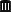Internet Archive.↩︎

6. Health Canada, HPFB, Guidance for Industry. Conduct and Analysis of Bioavailability and Bioequivalence Stu­dies - Part A: Oral Dosage Formulations Used for Systemic Effects. H42-2/56-1992E. Ottawa. 1992. Online.↩︎

7. Stupid example: CV = 10% (NTID), n = 120, 4-period full replicate design, $$\small{\theta_0=98.5\%}$$
→ 90% CI: 97.03 – 99.99%, $$\small{p}$$ 5·10–72↩︎

8. Schütz H. Sample Size Estimation in Bioequivalence. Evaluation. BEBA Forum. 2020-10-23. Online.↩︎

9. Berger RL, Hsu JC. Bioequivalence Trials, Intersection-Union Tests and Equivalence Confidence Sets. Stat Sci. 1996; 11(4): 283–302. JSTOR:2246021.Open Access.↩︎

10. Zeng A. The TOST confidence intervals and the coverage probabilities with R simulation. March 14, 2014. Online.↩︎

11. EMA, CHMP. Tacrolimus granules for oral suspension 0.2 and 1 mg product-specific bioequivalence guidance. EMA/CHMP/159744/2016. London. 31 April 2016. Online.↩︎

12. Mohanty L, Bhushan S, Rüttger B. Bioequivalence of two tacrolimus 1-mg formulations under fasting conditions in healthy subjects: A randomized, two-period crossover trial. Int J Clin Pharmacol Ther. 2020; 58(3): 183–93. doi:10.5414/CP203534.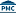Free Full Text.↩︎

13. Given, I estimated 54.↩︎